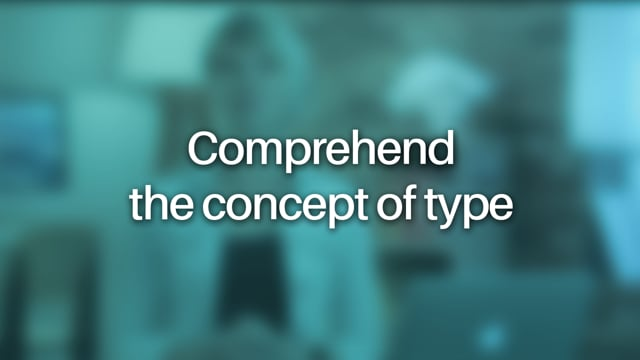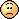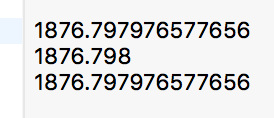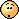Mis à jour le 06/03/2020

## Comprehend the concept of type

Connectez-vous ou inscrivez-vous gratuitement pour bénéficier de toutes les fonctionnalités de ce cours !We've just learned the essence of a variable, which is assigning a name to a value (labeling some data/information). We can also perform simple operations with variables using basic operators. However, not everything went so smoothly. Remember this code?

``````3/2 // the result is 1
3/2.0 // the result is 1.5
``````

Why is this the case?

Indeed, it's not clear. Let's investigate!

Now it's time to complete the picture about variables.

A variable is made up of three components:

• Name

• Value

• Type

Type indicates what kind of data something is, or, the value of a variable. For example, it could be a whole number, like 1, or a decimal number, like 1.5 to reflect our code example above.

Here are a few examples of type:

• Integer

• Decimal

• String

• etc.

What is the benefit of types?

Types provide two benefits:

1. Selective treatment. When a variable is used, since it has a type, the computer will know what it is and will be able to choose a suitable method to treat them. Here are a couple of examples:

• If we are using  `+`  operator on numbers, the result will be a numeric calculation (  `2 + 2`  => 4 ). This is the opposite for strings (  `"Hello, " + "World!"`  => "Hello, World!") where the expected result will be a composite string and not some mysterious mathematical interpretation.

• If we'd like to apply a specific action to a string, such as making it uppercase, we can do so.  This kind of action is not available for numeric types.

2. Correct storage in memory. Specifying type allows us to store the variable correctly in memory. For example, an integer and a decimal number require different sizes of memory. The computer is able to allocate the exact amount of space necessary for each variable by recognizing the type and thus ensuring data integrity.

Numbers in Swift can be one of three types, using the following keywords for declaration:

• `Int`  to declare an integer

• `Float`  to declare a decimal number

• `Double`  to declare a decimal number

Hm... isn't it the same thing twice?

Well noted! There are 2 different types to declare a decimal number:  `Float`  and  `Double`. Why? Precision. As the name Double suggests, it's twice big as Float.

Meaning what exactly?

This means that a  `Double`  variable can store 2 times more precise numbers than  `Float`.

And what does that mean?

You are unstoppable! Let's look at an example. We need to create a variable to hold the value`1876.79797657`. It is a very precise value! It requires 12 digits!`Double`  can store it in memory, because this type provides enough room for 12 digits.

`Float`  will only store the first 6 digits, as it has half the space of  `Double`. It will therefore store:  `1876.79`.

Meaning?

Meaning that we lose some important information. It's like if we dumped lots of stuff in one of our drawers without realizing it won't fit.

And what are bits now?

A bit is an elementary memory cell in a computer that can accommodate only one of two values: 0 or 1.

8 bits as a group then form what's called byte

And some more:

• 2 bytes form what's called a word

• 2 words (4 bytes) - a double word.

• 1024 bytes - a kilobyte (KB).

• 1024 bytes - a gigabyte (GB).

To sum it up for a reference:

 #of bits #of bytes Bit 1 - Byte 8 1 Word 16 2 Double word 32 4 Kilobyte, KB 8,192 1024 etc.

Applying this to the numeric types in Swift we just learned (generic):

 #of bits #of bytes Int 16 2 Float 32 4 Double 64 8

No more details! Now back on track!

So, which type to use to store a decimal number?

It depends on the precision you want to get. In practice, if the two solutions may be suitable Apple recommends using `Double`. A floating point number is also evaluated as a  `Double`  by default by Swift. This ensures that no information is lost, just in case.

Use  `Float`  only if Swift requires it or if you have an exceptional need for performance.

Swift requires that a variable always has a type. Swift is able to infer the type using its assigned value, in which case it's not necessary to explicitly specify the type. At times, however, we're going to want to explicitly specify the type. Here's the syntax for type specific declaration:

``````var name: Type = value
``````

For example:

``````var height: Float = 180.5
``````

This code declared a variable named  `height` , type  `Float`   and value  `180.5` .

To better understand the implications of this, let's look at this code (copy it to your Playground file):

``````var a:Double = 1876.79797657765609870978709780987
var b:Float = 1876.79797657765609870978709780987
var c = 1876.79797657765609870978709780987
``````

The code explicitly declares  `a`   as  `Double` ,  `b`   as  `Float`  and  allows the program to infer the type of  `c`   by its value. Here's what the Playground output looks like:We can observe a few things for each respective line:

1. The number is too large to be stored in full even for a  `Double`  type. It is therefore truncated after the 16th digit.

2. The variable is indeed a  `Float` since it's truncated to half the length of variable  `a`   which is  `Double`.

3. Swift has inferred a `Double`  type by identifying the decimal point in the value. `Double` is the default type for decimal numbers.

Enough about decimals (Float  & Double), let's not forget Integers (Int) that stores the whole numbers. And remember how our code looks like:

``````/* initial data */
// price of the purchase
let price = 1499

// daily savings allowance
let dailyAllowance = 10

/* calculations */
// days to save
let savingDays = price / dailyAllowance
``````

The variable  `savingDays`  is an integer, therefore of type  `Int` . However, it is expected that the result of 1499/10 will not be a whole number. We will therefore modify our declarations in order to enable a non-integral result.

``````var price: Double = 1499 // or alternatively => var price = 1499.0
``````

After this change, the Playground presents an error. Indeed, the variable    `price`   is now  `Double`  but the variable  dailyAllowance is still an integer.

To correct this, we need to declare  `dailyAllowance`   as  `Double` . Do this on your own and observe that  `dailyAllowance`   now contains a decimal number.

The `String`  type in simple terms represents a set of characters (i.e. text - phrases and words).

In order for the computer to distinguish between code and a string value, we must use quotes:

``````"I am a string"
``````

And, of course, we can declare a variable that contains a string. In this case, Swift will infer its type:  `String`.

``````var text = "I am a string" // Swift infers the type of the variable text
``````

Or, we can also specify the type explicitly just like for the numerical types:

``````var explicitText: String = "I am definitely a string"
``````

In practice, you will unlikely need to do this type of explicit declaration as there's no way for Swift to misinterpret it.

Ok, what can we do with it?

Like for numeric types, we can use operators for strings and reassign those modifications. The first way to modify strings is by concatenation.

What's that?

The concatenation is connecting two or more strings together - one after the other. This is done using the  `+`  operator. For example :

``````var phrase = "I am the beginning... " + "And I am the end!"
// Playground outputs: "I am the beginning... And I am the end!"
``````

Simple, isn't it?

Let's complicate things a bit and try to include a number in a sentence:

``````var numberOfMeals = 2
``````

With this information, we could build a sentence:

``````"I eat 2 meals a day!"
``````

To compose a dynamic sentence we need to replace a static number 2 in our sentence with a value in  `numberOFMeals`   variable:

``````"I eat \(numberOFMeals) meals a day!" // Playground outputs: "I eat 2 meals a day!"
``````

All we needed to do was to put the  `numberOFMeals`  variable in parentheses and add the backslash in front.

Let's do a little exercise! Try to write the following message in your playground project: "Dear Jenny, you'll have to save for X days to pay \$Y for your new coffee station.". Where X and Y are values of related variables we previously declared. Try on your own!

``````// Try it on your own before scrolling to see the result!

let message = "Dear Jenny, you'll have to save for \(savingDays) days to pay \$\(price) for your new coffee station."
``````

Got it? Congrats!

With this new technique, we will be able to generate our message to Jenny directly from the Playground!

Nothing major, just to sum up what we've already learned.

Swift was designed to be particularly safe for programming, to protect against potential errors especially those that are likely to come up at the time of execution of an application. This makes Swift highly structured. It helps developers to avoid unnecessary confusion due to misinterpretation of their intentions. This is especially true for types. Unlike some other languages, Swift is very strict with variables and imposes two rules:

#### 1. A variable can not change its type

Let's try to change it:

``````var text = "I am a string" // the type is String
text = 2 // to hold a number, the same variable must convert to Int type
``````

Swift generates an error: Can not assign value of type 'Int' to type 'String'. Oops.

#### 2. A variable always has a type

In other words, a variable without type does not exist. We cannot write the following code:

``````var someVariable
``````

Swift prompts an error: Type annotation missing in pattern

On the other hand, we can declare a variable with no initial value:

``````var someVariable: String
``````

This type our variable has a type: Swift is content! That's all it needs. It can manage the value assigned  later.

• A variable is a composition of three components:

• Name

• Value

• Type

• There are 3 ways to declare a variable:

`````` var text: String
// The type is annotated, which is necessary because the variable is not initialized with a value
var text = "I am a string"
// Th type is inferred from the value
var text: String = "I am a string"
// The type is annotated, it is not necessary because it can be inferred. But this is not prohibited
``````
• You now know four types:

• 3 numeric types:

• `Int`  or integers

• `Float`  or decimal numbers

• `Double`  for decimal numbers with 2 times more precision than float.

• 1 type for strings:  `String`

• We can concatenate the strings with using operator `+`

• A variable of another type can be inserted with `\ (differentVariable)`.

• Swift imposes some strict rules:

• A variable always has a type

• A variable can not change type after declaration